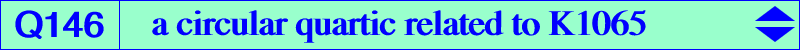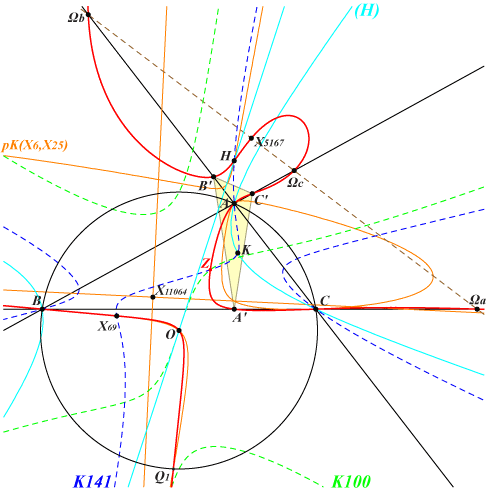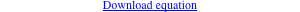too complicated to be written here. Click on the link to download a text file.X(3), X(4), X(69), X(2574), X(2575), X(5000), X(5001), X(5167) vertices of the orthic triangle A'B'C' centers Ωa, Ωb, Ωc of the Apollonius circles other points belowQ146 is a quartic related to the mapping 𝚹 : P → aQ described in page K1065. It is indeed the locus of M such that the orthocenter X(4) of ABC, P and 𝚹(P) are collinear. It follows that Q146 must contain the 6 singular points and the 7 fixed points of 𝚹, among them Z always real. Q146 is circular with isotropic tangents meeting at X(11799) and two other real asymptotes parallel at X(11064) to those of the Jerabek hyperbola. Q146 meets the Euler line at X(3), X(4) and two other points (imaginary when ABC is not acute) X(5000), X(5001) that lie on the circum-hyperbola (H) passing through X(6), X(232), X(250), X(262), X(264), X(325), X(511), X(523), X(842), X(1485), X(2065), etc. See also Q147.